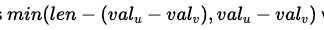# Need help in CSES - Planets Queries II

The provided solution states the following for the cycle case:

My question is that because we are given a directed graph, shouldn’t there only be 1 way (if possible) to go from vertex u to v? Why do we need to do the following?My understanding is that the general cycle case is that from u to v, we need to cross a cycle. So the distance is consist of 3 parts.

1. depth of u is from u to its root vertex that is inside the cycle;
2. depth of v is from v’s root vertex that is inside the cycle to v;
3. from u’s root vertex to v’s root vertex. Here there should be only 1 possible direction because all edges are directed.

I assume that the root of u is the 1st vertex that is in the cycle that we’ll run into if we traverse from u. Similarly the root of v is the 1st vertex that is in the cycle that we’ll run into if we traverse backward from v. Maybe this is an incorrect assumption?

Nah, I read the code, and you’re correct. Another incorrect thing about the solution is in the tree case, where it says you need to calculate “depth(a) + depth(b) - 2 * depth(lca(a,b))”, when all you needed to do is check if b was the ancestor of a, and calculate depth(a) - depth(b). I think the provided solution assumed that the graph was indirectedAhhh, I see. I thought I missed some critical information so that I can’t understand the explanationThanks for your time. I’ll wait for this to get updated. @Benq Thanks for starting a pull request.